LEONARDO DA VINCI ‘S DRAWING

THE MAN CIRCUMSCRIBED BY THE CIRCLE GENERATES THE GREAT PYRAMID'S GEOMETRY

NEW DISCOVERY: THE MAN CIRCUMSCRIBED BY THE SQUARE GENERATES THE GEOMETRY

OF THE PYRAMID OF MICERINUS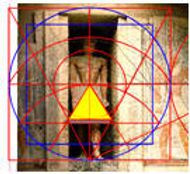NEW FINDINGS AND DISCOVERIES

DISCUSSED BY THE AUTHOR

IN HIS BOOKS

NEW DISCOVERY:  THE MAN CIRCUMSCRIBED BY THE SQUARE GENERATES

THE PYRAMIDAL MEN’S SKIRTS CUSTOM USED BY THE EGYPTIANS

NEW DISCOVERY:  EXAMPLE FROM MERERUCA’S TOMBTHE  GREAT PYRAMID AND MICERINUS STRUCTURE IS ENCLOSED

IN THE PERFECT SYMBOL (PROPORTIONAL DRAWING)

APPLICATION OF GEOMETRY  TO DETERMINE HUMAN'S HEIGHT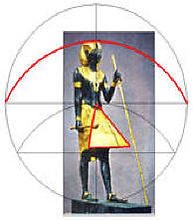PYRAMID’S CAPSTONE (BEN-BEN)

PYRAMID’S CAPSTONE (BEN-BEN) - DESIGN OF TOP STONE FOR PYRAMIDSDESIGN OF THE ORIGINAL ENTRANCE TO THE GREAT PYRAMID

BY SAMUEL LABOYPYRAMID’S ORIGINAL ENTRANCE                                      DESIGNED ENTRANCE

DESIGN OF THE KING’S CHAMBER  AND ITS FIVE CEILINGS

BY: SAMUEL LABOYDESIGN OF THE KING’S CHAMBER  AND ITS FIVE CEILINGS

DESIGN OF THE GRAND  GALLERY BY SAMUEL LABOY

DESIGN OF LONGITUDINAL SECTIONGRAND GALLERY - DESIGN WIDTH - CROSS SECTION

QUEEN’S CHAMBER AND ITS “NICHE”THE QUEEN'S CHAMBER                         Details of the "niche"

QUEEN’S CHAMBER - LOOKING EAST            EAST WALL AND  “NICHE”

DOUBLING THE CUBE IN THE PYRAMIDS... ? YES...

SEE HOW...THE EGYPTIAN PYRAMID’S BUILDERSTHE MOST INTERESTING PYRAMIDS...

Why the Bent Pyramid in Dahshur, Egypt, is bent?BENT PYRAMID

(Designed by Samuel Laboy)

The Bent Pyramid was built in Dahshur, Egypt, by pharaoh Sneferu, father of Pharaoh Khufu, builder of the Great Pyramid. The Bent Pyramid is better known by its two different angles in its inclined sides. Egyptologists indicates that this Pyramid was under construction, when its initial angle had to be changed; up to the pyramid’s top, as necessary to repair a faulty design of the Egyptian engineers, or by the failure of the structure.

For Engineer Samuel Laboy, from Puerto Rico, this is a simplistic solution which buries well underground one of the most important and historic pyramids design in Egypt. To solve this design, he used the Perfect Symbol system, previously used by him to solve the geometric design of the Great Pyramid, and others. He found that the Bent Pyramid shape is composed of the superimposition of two important and unique pyramid’s designs. He explains that the reasons for the pyramid combined design could be, from the spiritual point of view of the Egyptians, to provide with this new geometric arrangement, a powerful and energetic force to the pyramid.

As a professional Civil Engineer, Laboy do not accept the theories elaborated to explain the changes in the angles. He believes the pyramid was designed and built as iconstructed. He said that the two geometric configurations, when superimposed, using an equal scale, create a combined configuration exactly equal to that of the Bent Pyramid.

The following is the method Engineer Laboy used to rescue and to determine the Bent Pyramid’s design. He states that this is not a theory, if he said so, he would be lying. The fact that all calculations, properties and characteristics, exactly fit the design, like he did with the Great Pyramid geometric design, is irrefutable evidence of it exactness, and proves that this is another very important discovery for the Egyptology world.

As he explained, demonstrated and illustrated in his new books “A Civil Engineer looks at the Great Pyramid” and its “Supplement”, the Bent Pyramid follows a geometric pattern composed of two completely different geometrical configurations. Each configuration has a particular reason to be incorporated in the Bent Pyramid’s design.

The first model establishes the lower angle of the Bent Pyramid. Its configuration represents the pyramid that is created with the well-known configuration of a square circumscribed by a circle. He said that this would be the simplest pyramid’s design model of a pyramid. The tangent of the slope of this pyramid is equivalent to the square root of two (√2). Therefore, the corresponding angle for this slope is 54° 44’ 8.197”, and as it is known, this is the correct angle for the lower section of the Bent Pyramid.

The second pyramid’s model, when superimposed over the first, shows the upper angle of the Bent Pyramid. This pyramid has an important and special configuration. In this case is one of the most elaborated, complicated and amazing configuration for a pyramid. It seems that the designer is including in the Bent Pyramid’s design the complete range of pyramid designs, from the simplest to the most elaborated. Laboy explained that he classified this pyramid in his main book, in Chapter 10, as type VI, the special case, “when the construction circle is located inside the base of the pyramid”. In this particular case, the perimeter (P) of the pyramid’s base is equal to the diameter of the circle multiplied by Phi cubed (=D
f³). When the cross sectional views of both model pyramids are superimposed under equal scales, the cross sectional view of the Bent Pyramid, emerges.

f = 1.618033989..., is given by our Creator as a proportioning parameter in the micro and macro cosmos. It appears in human proportions, as illustrated by Leonardo Da Vinci in his famous human proportion’s drawing, in some animal bodies-shapes, even in the shapes of the expanding galaxies in the cosmos. Its decimal numbers never ends, as the function of Pi = p = 3.1415926…, .

The Phi function number (f) is easy to construct using mathematical series, from a square figure, even from a circle. He discovered that the right triangle with sides in the proportion 1:2 is a (Phi function generator). This simple triangle, easily set with three nails and a chord, will provide the solution, or answers, to practically all (f) combinations. This method could have been the ancient (Egyptian-computer) to work out the f functions. However, as known, and experienced by me, Egyptologists have banned the Phi function, and the circle configuration from the pyramid’s studies, and what are really are doing is closing the entrance doors to the pyramid’s geometrical solution.

As a side note, apparently, the Phi cubed (= f³) was very important for the Egyptian  works. It was used to build the Red Pyramid and the top of the Bent. This relation is so important in geometry and Maths, that there is no doubt that the Egyptian designers knew about it and used it to strengthen, or give power to their construction works.

The ratio of the circle’s diameter and the pyramid’s side length (= D / b),  represents the function of the tangent of the angle of the face for a pyramid. For example, in the upper section of the Bent Pyramid, this ratio is equivalent to 4 divided by (
f³) = 0.94427191. Therefore, the angle corresponding to this tangent’s function is 43° 21' 29.51", exactly the same angle measured at the upper section of the Bent Pyramid.

From another point and very important, apparently, Pharaoh’s Chephren knew very well about the two angles which formed the Bent Pyramid's structure, He considered them so important that for his pyramid in Giza, he used the combination of the two slope angles which  composed the Bent pyramid's structure. Therefore, he multiplied the (square root of 2) = (2)corresponding to the lower section, by 4 divided by (f³) for the upper section. The resulting slope is1.335402142, which gives the angle 53.17273225° = 53° 10’ 21.83. This is the angle used for his pyramid at Giza.

From another point, Engineer Laboy found and explains in his books how to set the descending passages for the pyramids. Using his geometrical method and the Perfect Symbol, he designed, independently, the descending passage of each of the two pyramid's models of the Bent Pyramid. Therefore, both passages were also superimposed in his Bent Pyramid’s geometric design. He found that the cross section of the pyramid corresponding to the lower section of the Bent Pyramid was set from south to north, therefore, the entrance door is at the north; while the pyramid’s design for the second pyramid, was superimposed from east to west, therefore, the entrance door is located at its west side. Both his designed descending passages corroborate the two descending passages lengths and elevation built for the Bent Pyramid. It is remarkable that the designed passages by Laboy and and the constructed at the Bent Pyramid’s structure, illustrate the same entrance location and elevation.

Laboy concluded that the superimposition of one pyramid's model design, over the other, creates the two angles shown by the Bent Pyramid. In his model, the vertical distance from the pavement to the change in angle elevation, was calculated as 154.60 ft. As a matter of fact, this is the vertical distance measured at the Bent Pyramid's structure between these two points. In addition, the descending passages and entrance doors, and other calculated dimensions, angles, and lengths are exactly equal to those measured by surveyors at the Bent Pyramid’s structure. We do not need an old papyrus to tell us that his designed plan corresponds to the Bent Pyramid. He said: "Egyptologists can read hieroglyphs, Engineers can read structures".

It is interesting to examine the special case of the Red Pyramid and the upper section of the Bent Pyramid. The ratio between the circumference of the circle and the perimeter of the square (C / P) is equivalent to (p / f³). Note that the perimeter of the square base is equal to the diameter of the circle multiplied by f³, or P = D f³, and also that the radius R = 2 (b) / f³.

That is, the ratio (C / P) = p D / 4 (b) = p D / P = p D / D f³, which finally shows that (C / P) = (p / f³). Having (C / P) = (p / f³), it can be established that the perimeter (P) of the square base for this pyramid would be equal to the diameter (D) of the circle, multiplied by f³, that is, P = D f³, and that R = pyramid’s height = 2 (b) / f³.

I am sure that all of these math's and geometry can be understood by Egyptologists, or can be referred to their favorite Universities for verification. They are irrefutable evidence of the great knowledge in those sciences of the Ancient pyramid builders. We should not negate this ancient Culture their great knowledge in this sciences because they did not left and easy to find papyrus,  to prove it. However, they left their extraordinary works to prove that they did them.PROPERTIES OF THE DESIGN OF THE RED PYRAMID

DESIGN OF RELIGIOUS AND

ORNAMENTAL OBJECTS

using the Perfect SymbolTHE ZODIAC OF DENDERA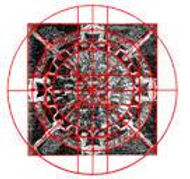DESIGN  OF THE ZODIAC OF DENDERA    ZODIAC

FROM THE BOOK:

A CIVIL ENGINEER LOOKS AT THE GREAT PYRAMID "SUPPLEMENT"

Important information missing from Leonardo’s human proportions drawing!

Leonardo Da Vinci’s drawing about the human body proportions (also called de Vitrubius man) illustrates the figure of a man superimposed in two different positions, one circumscribed by a circle, and the other by a square. He did not mention that they represent the geometry of the Great Pyramid and that of Micerinus.

Leonardo wrote in reference to the man’s positions; ‘If you set your legs so far apart as to take a fourteenth part from your height, “and you open and raise your arms until you touch the line of the crown of the head with your middle fingers, you must know that the center of the circle formed by the extremities of the outstretched limbs will be the navel, and the space between the legs will form an equilateral triangle”. For the man’s second position he indicates; that when the man with his arms extended horizontally and his legs and feet are closer together, the spans of his outstretched arms are equal to his height and his body will be circumscribed by a square.

He wrote that this canon of proportions was one of the parameters that the Creator used to proportion the human figure, but that if applied in every person it would be difficult to distinguish them. This traditional canon of proportions for the human figure provides a system of mathematical and geometrical relationships to represent the anatomical structure of the human body.

Leonardo illustration was based on a passage of the Ten Books of Architecture published by a Roman architect named Marcus Vitruvius; where he explains that the human body can be circumscribed by both a circle and a square. Leonardo’s drawing was sketched to illustrate the Golden Proportion (or f function) in a book to be published at those times by a mathematician known as Lucca Pacioli. However, the knowledge about these properties and characteristics of the humans, shown by Vitruvius and Leonardo, could have had its origin in the ancient Egyptian Culture.

Engineer Samuel Laboy, from Puerto Rico, who designed the entire geometrical plan of the Great Pyramid, and revealed its secrets and dimensions, also discovered missing important information from Leonardo’s drawing. He proved and evidenced that Leonardo’s drawing is based on a section of a geometric symbol, that the ancient Egyptians used as a design pattern for their arts, temples, statues, and pyramid’s designs. This symbol, which Engineer Laboy reinvented about 4 decades ago, and called the Perfect Symbol, permitted him to design the Great Pyramid and many other pyramids, religious temples, art-jewelry, and many other Egyptian constructions designs. All of these is evidenced in his Report books “A Civil Engineer looks at the Great Pyramid, and its Supplement.

He clearly demonstrates that Leonardo’s drawing was derived from his Perfect Symbol. Although Leonardo sketched a modern looking man in his drawing, it is most probable that the original drawing, or geometric idea, could have illustrated an ancient Egyptian man, in Egyptian times.

When he examined Leonardo’s drawing using the perfect Symbol, he discovered some important hidden information in his drawing. It is something that Leonardo did not seem to have noticed, or mentioned in his writings. This information concerns the ancient Egyptian culture, known as the Pyramid Builders. This data, clearly identifies the Great Pyramid and the Pyramid of Micerinus.

Leonardo’s drawing could be better explained using the Perfect Symbol since it represents a section of the symbol’s geometry. Leonardo’s drawing is really a section of the Perfect Symbol and shows all the perfect human dimensions, combined with the perfect pyramid. These three perfect figures are so important for the mankind that he decided to call this new improved symbol, the Universal Symbol. The Universal Symbol, drawn entirely with a straightedge and a drawing compass, shows the construction circle, the triangle (cross sections) and the two squares bases of two pyramids at the Giza Plateau.

The figure corresponding to the man’s circumscribed by the circle, shows the square of the base of the Great Pyramid and its characteristics and properties, the other figure corresponding to the erected man and closed feet, represents the base and characteristics of the pyramid of Micerinus.

Going further about this Universal Symbol, the proportional cross section corresponding to the Pyramid of Micerinus identifies what clearly be interpreted as the origin of the pyramidal men’s skirts fashion, used by the ancient Egyptian men. All this information is missing from Leonardo’s drawing. These important discoveries link his drawing with the ancient Egyptian times. These findings never have been exposed before by anyone.

If the radius of the circle is set equal to 1, the entire Universal drawing can be calculated. If the radius is set equal to the pyramid’s height, the triangle will exactly represent the Great Pyramid’s cross section and angles, the cross section of the pyramid of Micerinus dimensions and angles. This information is completely exposed and evidenced in the Author Report-books.

As can be seen, Leonardo drew a numerical scale at the base of his drawing, where he sets the value of f as the side length of the square, equivalent to 24 units. Therefore, we have to assume he drew his drawing base on this scale. On the contrary, the universal symbol, created by Engineer Laboy using just a straightedge and a drawing compass, permits us to perfectly draw his drawing using no numbers, scales, or mathematics calculations. All corresponding figures are shown in his Report Books.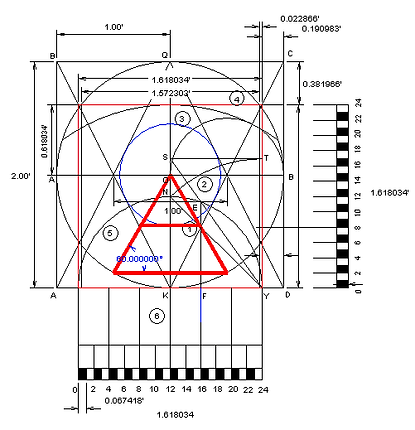If the radius of the circle is made equal to (1.00 unit), the diameter is equivalent to (2.00). The horizontal numerical scale shown in Leonardo's drawing represents the f function = 1.6180339 = 24 units shown at the base. The vertical f scale, illustrated by Laboy completes Leonardo's square.

DECODING THE GREAT PYRAMID'S CONFIGURATION AND LEONARDO'S DRAWING

USING THE PERFECT SYMBOLI remodeled Leonardo’s drawing to show his man's figure which shows and establishes the cross section of the Great Pyramid. If the radius of the circle is made equal to one (1), called a unitary circle, the entire figure can be accurately calculated. However, the radius can be any number using any measuring units.  To obtain the Great Pyramid's dimensions in feet, set the radius equal to (153 times π) = 480.6637 ft. If you want meters, use 146.50807 meters. If Egyptian cubits, use 279.5920 cubits of 20.63 inches. The results will be expressed in the units used for the radius. In other words, the drawing is a design template.

The calculated angles of the sides of the pyramid are 51.827293°. Note that the completed design of the pyramid includes the triangle PQN, which illustrate the important underground area of the pyramid, established between points PHTN.

From the other part, the man’s circumscribed by a square establishes the characteristics and properties of the Pyramid of Micerinus (cross section of triangle between points (OSL). The blue triangle between points (b), (a) and (O) set the angle 51.026554° = 51° 1’ 35.59” between the corners of the square set by points (a, b, c, and d). This angle corresponds to the Pyramid of Micerinus. The circle tangent to the inclined blue lines establishes the pyramid’s height as 214.9593, and the base of the pyramid as 347.62.48 ft. It is important to note that the dimensions of the pyramid of Micerinus are derived using the radius as 480.6637 ft.

The internal design of the Great Pyramid, as established by the Perfect Symbol, shows us the spiritual reasons for their existence and locations of its sections. They superimposed the geometric concepts and parameters used by our Creator with the human existence with the pyramid's structure. Observe that the man's navel represents the center of the circle which circumscribed the man. For the humans is the chord that extends life continuance. For the pyramid represents the center of the base of the Great Pyramid.

The underground center of the base of the pyramid, at point X, showing the Phi = f point location, corresponds to the genitals location, which denotes  man's reproduction and satisfaction, eternity, pleasure. This is the perfect location for the real pharaoh's Mortuary chamber. It is the most important location of the entire figure. The ascending passage and the Grand Gallery permits to elevate the Pharaoh's soul to the King's Chamber elevation, which corresponds to the man's heart elevation in the human figure. Then, through the antechamber it moves horizontally to inside the King's Chamber, where the sarcophagus is located. It is like Like the soul looking for the human's heart location. Therefore, it makes sense the comment that the sarcophagus in the King's Chamber vibrates at the same frequency as the human heart.

The combination of the three figures, the perfect Symbol, Leonardo's drawing, and the Great Pyramid's geometry, lead us to think about a secret spiritual connection between the three symbols.

Somehow Leonardo, like a Super human he was, to use his drawing as a means to stimulate humans to free and clean his soul to enter unknown spaces, which will provide the "real peace and security. You can use as reference, the new and extraordinary book "The Super Human Effect" by Dennis Rodriguez.

The Super Human EffectAuthor: Dennis Rodriguez

As the figure of the man in Leonardo's drawing, when circumscribed by the circle represents the Great Pyramid, and when circumscribed by the square, represents the Pyramid's of Micerinus, Author Rodriguez added the wings to Leonardo's man's drawing, representing his search and quest for the moment when everything changes.

POSSIBLE EGYPTIAN DRAWING WHICH MAY

HAVE INSPIRED VITRUVIUS AND LEONARDO, TO DRAW A SIMILAR SKETCH

The following figure, sketched by Engineer Laboy, suggests a possible Egyptian  drawing of the human figure, using his discovered geometric Symbol, which exactly set the geometry of the Great Pyramid, and that of Micerinus, in Leonardo's drawing.

As known, besides the Great Pyramid, and that of Micerinus, we find the Pyramid of Chephren in the Giza's area. There is a notable correlation between these two Giza's pyramids, and the Bent Pyramid at Dahshur in Egypt. As stated, for the Bent Pyramid, the slope angle of the lower section is defined by 2, where its angle is represented by the slope  1.414243562, or 54.73561032° = 54° 44' 8.197". The upper section angle is defined by (4 / f³) = 0.94427191, for an angle of 43 21' 29.51".

From another side, The slope of the pyramid of Chephren corresponds to the product of the two different slopes which composes the Bent Pyramid's design. In other words, (2)(4/f³) =  1.335402142 slope, which indicates an angle of 53° 10’ 21.84”. This angle corresponds to Chephren's Pyramid angle.

It can also be said that the square root of two (=2) multiplied by ratio between the area of the base of the Great Pyramid and Micerinus, equal to (4 / f³),  also represents the slope angle of the Chephren Pyramid.THE UNIVERSAL SYMBOL

POSSIBLE EGYPTIAN DRAWING ORIGINATING

LEONARDO'S HUMAN PROPORTION SKETCH

Concept and drawing by: Samuel Laboy

DISCOVERY:   1. THE MAN'S FIGURE CIRCUMSCRIBED BY THE CIRCLE

WILL SET UP THE GREAT PYRAMID'S GEOMETRY

DISCOVERY:   2. THE MAN'S FIGURE CIRCUMSCRIBED BY THE SQUARE

WILL SET UP THE PYRAMID OF MICERINUS GEOMETRY

THE PYRAMID OF MICERINUS

IN LEONARDO’S DRAWING

Observe that there is a small difference in the horizontal span distance between the ends of the middle fingers of the man touching the circle = (2 / √f), and when he is touching the vertical sides of the square = f. They correspond to the sides of the square (drawn by Leonardo) and the other added by engineer Laboy.This small difference creates the data between the Great Pyramid and Micerinus.THE MAN'S FIGURE CIRCUMSCRIBED BY THE SQUARE:

SET UP THE PYRAMID OF MICERINUS' GEOMETRY

This small differences between the two spans, changes the triangle’s slope formed when a line is set between the top of the circle (Q) to the point of intersection of the projection of the vertical sides of the squares in the horizontal diameter.

For the Great Pyramid cross section, the inclined line from point Q goes to point H and T. This geometric arrangement exposes the information needed to design the Great Pyramid.

On the other hand, a similar condition exists for the inclined lines traced from point Q to the intersection between the horizontal diameter and the vertical lines, corresponding to the square with f sides. To facilitate the drawing, the center O of the circle will be used instead the Q point, but is equal. The two inclined lines will be traced from point O, to point (a) and to point (b), as shown in the next figure. The angle of these two inclined lines corresponds to the pyramid of Micerinus. However, when from point O is traced a circumference tangent to lines UK and VK, that the height and the base length of the Pyramid of Micerinus is revealed.

I named this extension of the Perfect Symbol as the Universal Symbol, shown it the next figure. As shown, in the diagrams the Universal Symbol can be resolved using units of f, using units of numbers, or any other units of measurements desired by the user. For the example in the figure the radius is equal to 1 and the diameter = 2. To calculate the desire section, multiply the formula by the desired radius for the circle.

THE KEY ARCHITECTURAL TEMPLATE FOR EGYPTIAN DESIGNS, AND MANY OTHER CULTURES IS THE ILLUSTRATED BELOW. IT WAS CREATED, DESIGNED AND COPYRIGHTED BY SAMUEL LABOY, AND NAMED THE UNIVERSAL SYMBOL.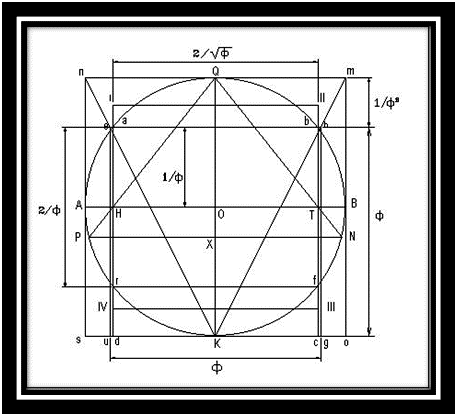THE UNIVERSAL SYMBOLTHE UPPER (RED) TRIANGLE DEFINES THE GREAT PYRAMID

THE LOWER (ORANGE) TRIANGLE DEFINES THE PYRAMID OF MICERINUSDIMENSIONAL REPRESENTATION OF BOTH PYRAMIDS

The face angle of the pyramids of the Great Pyramid and of Micerinus comes from the Perfect Symbol. However, that of the Pyramid of Chephren, represents the product of the two important pyramids which composed the unique design of the Bent Pyramid.

The next figure is an enlarged figure of the lower left section of the previous figure. In the next enlarged figure, the inclined red line crossing the horizontal diameter establishes the location of the vertical lines of the square corresponding to the man circumscribed by a circle. This same location establishes the width of the square, determined as (D / b) = (2) (2 / √f) = 1.5723028. This slope angle yields the angle 51.82729236 = 51° 49’ 38.25” The side’s length is b = (2 / √f) (480.6637) = 755.7488 ft. This information corresponds to the Great Pyramid.ENLARGED SECTION TO ILLUSTRATE

THE CHANGES IN ANGLES

The sides of the square, or span distance between the middle fingers of the man is equal to f. Its height = 1.0, the tangent of the slope is (D / b) = (2 / f) = 1.236067978, with an angle equal to 51.02655266° = 51° 01’ 35.59”. This angle corresponds to the pyramid of Micerinus.

Since the slope tangent is (D / b) = 1.236067978, then. D = (1.236067978) f = 2. For a pyramid's height of 214.95 ft, and a diameter D = 429.90 ft. The sides (b) of the square between points (1, 2, 3, and 4), correspond to (R) f  = (R) (1.6180339). For a pyramid’ height (R) = 214.95 ft., the side length would be 214.95 (f) = 347.80 ft, which represents the sides of the Pyramid of Micerinus.The red square in the above figure represents the base of the Great Pyramid, where its sides are equal to b = 2 (R) / √f. From the other part, the blue square represents the base of the pyramid of Micerinus, where its sides are equal to:   b = R (f).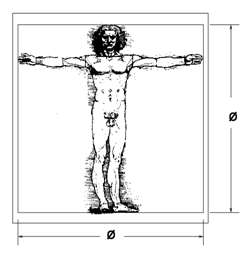PYRAMID OF MICERINUS SQUARE BASE =  (f³) (f³)

Therefore, it can be said that in Leonardo’s drawing, the figure of the man circumscribed by a circle,  represents the Great Pyramid, while when circumscribed by a square represents the Pyramid of Micerinus. The figure, illustrated below, shows the cross section of the Pyramid of Micerinus which is exactly equal to the men's skirt custom used by the Egyptians. Note that the navel of the man's figure establishes the top location of the skirt, exactly like that of the Egyptians.PYRAMID OF MICERINUS GEOMETRY

The base area of the Great Pyramid is (2 / √f)2 and the base area of the Pyramid of Micerinus is (f)2. Therefore the ratio between both areas is (2 /√f)2  /  (f)2 = 4 / f³. This number is equal to 0.94427191, which represents the tangent of the angle 43.35819755° = 43° 21' 29.51". This angle corresponds to the slope of the Red pyramid and the upper section of the Bent Pyramid.

From another point, the square root of two, multiplied by the ratio of the Great Pyramid base area and Micerinus, gives the slope angle of the Pyramid of Chephren,  (√2)  (4 / f³)= 1.335402142 = 53.17273225° = 53° 10' 21.84".

The properties of the angle of the Red Pyramid and that of the upper section of the Bent Pyramid is unique. For these pyramids. when the diameter of the circle (D) is multiplied by π, it gives the circumference of the circle (C = D (π), but when the same diameter is multiplied by f³ it gives the perimeter of the sbase of the pyramid (D f³) = P, = 4(b). Therefore, for this special pyramid, the ratio of the circumference to the perimeter D (π) / (D f³) = (π / f3³). This represents a special property for this pyramid. Thus, the side length of the base (b) = (D f³)/4 = (R f³)/ 2.

This is the slope angle of the Red Pyramid and of the upper section of the Bent pyramid. Therefore, Pharaoh's Chephren combined the two slopes of the Bent Pyramid for his pyramid at Giza. The √2 slope of lower section and (4 /f³) of the upper section = (√2) (4 / f³) = 1.335402142. The angle for this function is 53.17273225° = 53° 10’ 21.83”, angle which correspond to the slope of the Pyramid of Chephren..

As demonstrated and evidence, the bent of the pyramid is not created by a wrong design, or a building's failure, as believe by scholars. It is created from the superimposition of the designs of two different pyramids. These two pyramids have extraordinary characteristics and properties. The pyramid that set ups the angle of the lower section of the Bent Pyramid is created when the figure of a circle circumscribes a square. For this pyramid, its angle of the faces is represented by √2 = 1.414243562 or 54.73561032° = 54° 44' 8.197". This is exactly the angle measured at the pyramid.

In his writings, Leonardo Da Vinci did not mention the Perfect Symbol, or the Universal Symbol’s arrangement, nor the Great Pyramid, or any other figure that could be derived from his sketch, or which fit his drawing. We do not know, or never will know, if he knew about and never mentioned it, or he missed to find it. He drawing only shows the man’s superimposed double figure, one to be inscribed by a circle, and the other by a square.

LEONARDO'S CIRCLE AND SQUARE

Leonardo's circle and square were apparently drawn proportionally based on the numbered scale of 24 units as shown at the baseline of his original sketch. He sets the value of (f) = 24 units). This suggests that Leonardo made his drawing based on this "numbered scale". However Leonardo could have drawn the circle and the square by using simple geometry, as I will demonstrate in my next figure.1.  Draw a square of any size, as shown using points I, II, III, and IV.

2.  Divide line (III-IV) at its midpoint K and set the vertical line KQ and the inclined line K (II).

3.  Set a compass at point II, and using the distance (II-Q) as radius, draw the Arc QS. Mark point N at the intersection of line K-II with the arc QS.

4.  Set the compass at point K, and using as radius KN, draw an arc from one side of the square to the other side (mark points L, O, and M).

5.  Set the compass at point O, and using line OK as radius, draw the circumference of a circle.

6.  If the radius of the circle is one (R = 1), the sides of the square are equal to Phi (= 1.6180039). The formula is b = R (f), this formula corresponds To the Pyramid of Micerinus.

Therefore, the circle and the square arrangement shown in the drawing represent Leonardo’s figures of the circle and the square. He only needed to draw the man’s superimposed positions to complete his famous drawing.THE HUMAN'S FIGURE CIRCUMSCRIBED IN THE CIRCLE

IN LEONARDO’S DRAWING REPRESENTS

THE GREAT PYRAMID'S GEOMETRICAL DATA

Observe in the drawing that the inclined sides of the Great Pyramid, when extended to intercept the circumference of the circle below the base-line, creates the underground area below the pyramid, used for corridors and chambers. The location of point X establishes in the pyramid’s model the control point X (= f), which will represent in Leonardo’s drawing, the human’s genital reproduction organs. As a revealing fact, the human’s genitals represent the location of a Symbol of Reproduction, Eternity, Satisfaction, House of Peace, Power, and Control. At the same time, it illustrates the balance and control point for the whole pyramidal structure. I believe this would be the better selection for Pharaoh’s Khufu Mortuary Chamber construction. The man’s circumscribed by a circle reveals the characteristics and properties of identification of the Great Pyramid (the triangle HQT).

In the same drawing, the man’s circumscribed by a square reveals the characteristics and properties of the Pyramid of Micerinus (the triangle SOL). The triangle formed between points (HOT) exposes the cross sectional view of the pyramid of Micerinus, showing its top, at the man's navel.

GEOMETRIC PLANS IN   RELATION TO THE GREAT PYRAMID

AND THAT OF MICERINUSTHE GREAT PYRAMID AS A TRIBUTE TO MANKIND

The figure of the circle, the triangle, and the square coming from the Perfect Symbol, combined with Leonardo's drawing (the Egyptian representation) and the Great Pyramid's structure, clearly exposed the significance and existence of this Great Monument of the world, known as the Great Pyramid.

As it can be observed from the following composed figure, where I added the Great Pyramid showing its interior section, the geometrical and mathematical relations present enough data to build the mentioned pyramid at the Giza's  Plateau. Therefore, there is no doubt that the Egyptians engineers had known about the properties and characteristics of this drawing composition by the time the pyramids were built.Mereruka’s Tomb - The cross section of the pyramid of Micerinus

represents the men’s skirt fashion used by the Egyptians

PARAMETERS TO DETERMINE THE HUMAN’S HEIGHT

BY: SAMUEL LABOYTo determine, geometrically, the human’s height, based on any circumference, simply follow this procedure:

1. Draw a circle and trace its vertical and horizontal diameters.

2. Using a compass, from point K and distance KO as radius, trace an arc to cut the circumference at point (a) and (b).

3. Set a horizontal line from point (a) to point (b) and mark point N at its Intersection with the vertical diameter.

4. Set the compass at point N, and using the distance from N to A as radius, trace an arc from point A to point B, and mark point S at its intersection  With the vertical diameter.

5. The figure illustrates the vertical distance K to S, which is equal to f = 1.6180039, which represents the man’s height.

6. The vertical distance SQ represents (1 / f² ) = 0.3819660, or the circle’s free space over the crown of the man’s head. Therefore, the diameter of the circle is f (= 1.6180039) + (1 / f² ) (= 0.3819660) = 2.00. Using this procedure, the height of the man, painting, statue, etc. can be established, as shown in the next figures.

HUMAN HEIGHT’S PROPORTIONSHUMAN’S HEIGHT DETERMINATION USING GEOMETRY

DESIGN OF THE EGYPTIAN’S MEN'S SKIRT FASHION

BASED ON THE PERFECT SYMBOLEgyptians  men’s pyramid skirts -having its top at his navel